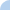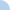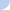# Check Your Understanding - The Force of Gravity

Choose the single best answer in each question. When you use the equation F = ma, if the mass is in kilograms, the acceleration is in meters per second squared (m/s2), and the units for force (F) are newtons (N). So 1 N is the force required to make 1 kg accelerate 1 m/s2.

Remember that the gravitational acceleration (g) on Earth is 9.8 m/s2.First name Last nameMs. Liu 6th Grade Science - CMS MA

 This activity was created by a Quia Web subscriber. Learn more about QuiaCreate your own activities